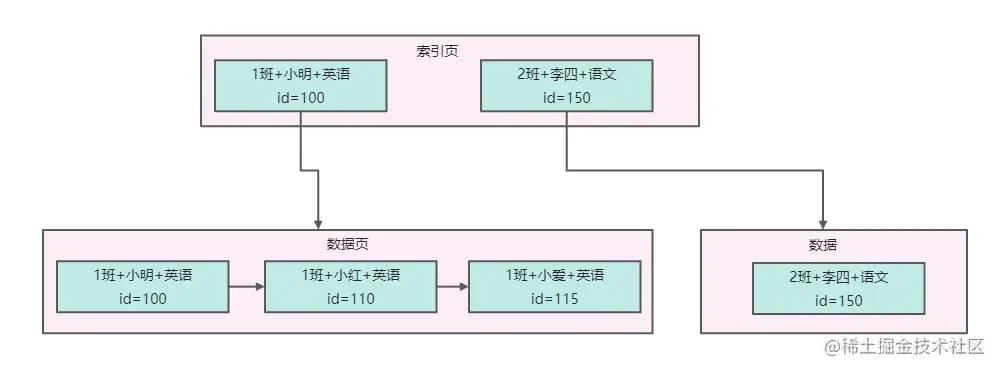# MySQL索引-索引匹配规则

2,025

「这是我参与11月更文挑战的第18天，活动详情查看：2021最后一次更文挑战# 最左侧列匹配

``````SELECT * FROM student_scope where class_name= '1班' AND student_name ='小明' AND subject_nane  ='英语';
SELECT * FROM student_scope where class_name= '1班' AND student_name ='小明';
SELECT * FROM student_scope where class_name= '1班' ;
``````

``````SELECT * FROM student_scope where class_name= '1班' AND subject_nane  ='英语';
``````

``````SELECT * FROM student_scope where student_name ='小明' AND subject_nane  ='英语';
SELECT * FROM student_scope where subject_nane  ='英语';
``````

# 最左前缀匹配原则

``````SELECT * FROM student_scope where class_name like '1%';
``````

``````SELECT * FROM student_scope where class_name like '%班';
``````

# 范围查找规则

``````SELECT * FROM student_scope where class_name >= '1班' AND class_name <'10班';
``````
``````但是当第一个字段是范围查询的时候，后面的第二个字段是没法走索引，例如下面的SQL,class_name 会走索引，后面的student_name 不会走索引。
``````
``````SELECT * FROM student_scope where class_name >= '1班' AND class_name <'10班' AND student_name ='小明';
SELECT * FROM student_scope where class_name >= '1班' AND class_name <'10班' AND student_name >= '小明';
``````

# 等值匹配+范围匹配规则

``````SELECT * FROM student_scope where class_name = '1班' AND student_name >= '小明' AND student_name <'王五';
``````

# 排序如何使用索引

``````SELECT * FROM student_scope ORDER BY class_name ASC,student_name ASC,subject_nane  ASC LIMIT 10;
SELECT * FROM student_scope ORDER BY class_name DESC,student_name DESC,subject_nane DESC LIMIT 10;
``````

``````SELECT * FROM student_scope ORDER BY class_name DESC,student_name ASC,subject_nane DESC LIMIT 10;
``````

# 分组如何使用索引

GROUP BY 语句使用索引的时候跟ORDER BY排序使用索引的规则一样，对于group by后的字段，最好也是按照联合索引里的最左侧的字段开始，按顺序排列开来，这样的话，就可以完美的运用上索引来直接提取一组一组的数据，然后针对每一组的数据执行聚合函数就可以。